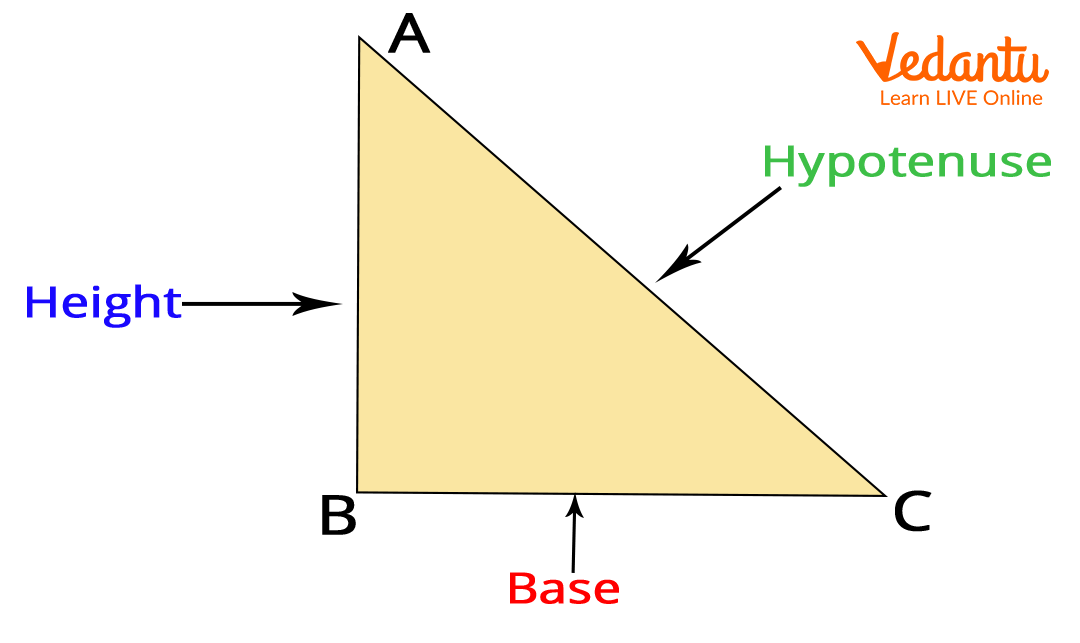Courses
Courses for Kids
Free study material
Free LIVE classes
MoreDo you know a number that starts from a letter ‘H’ and comes after counting of numbers from 1 to 99? So, what is that number? Yes, you guessed it right. It is H for ‘Hundred’. There are many other words that start from the letter H in Mathematics. These all words make a special space in Mathematics.

Here are some of the words that start from the letter ‘H’ that you must add up to increase your vocabulary.

## Mathematics Vocabulary of Letter ‘H’

1. Hundred: Hundred is a number that is written as ‘100’. It is a natural number and written as C in the Roman numeral.  It is the smallest three-digit number.

2. Half: Half is one of the two equal parts of something. Half of a hundred is 50.  If your mother gives you a pizza and asked to share it with your sibling equally. Then, you will divide a pizza into two equal parts, these equal parts are called two halves. (plural of half is halves.) Some of the common halves used in Mathematics are Half gallon, Half past, Half hour and half-circle.

3. Hexagon: H for Hexagon is a shape having 6 sides. Some shapes that start with H are Hemisphere, half-circle, Heptagon, Hyperbola. Can you think of any other shape that starts with the alphabet ‘H’?

4. Heavy: You can throw your ball easily, but can you throw the almirah? No? Try once. Yes, it is impossible to throw the almirah because it is H for ‘Heavy’. So, to compare the weight of two objects, we used the term H for ‘Heavy’.

5. Hour: If your cousin asks you how much time you spend in school, your answer would be 6 ‘hours’ a day. H for ‘Hour’, you used to define the time-span.

6. Horizontal:  When you grow a little bit, you will learn coordinate geometry. In coordinate geometry, you will learn about the X- and Y-axes. The axis which is parallel to the level ground is called the X-axis. In general, it is called the H for the ‘Horizontal’ axis. A horizontal axis is used to draw different bar graphs.

7. Height: Are you taller than your friend? No! Do you know why? Because your H for Height is less than your friend. Height is basically the measurement from the bottom to the top of a person or any object. Also, in geometry, H for Hypotenuse is the longest side of any right-angle triangle.1. Highest Common Factor: To find the largest number that divides two numbers is calculated by using the Highest Common Factor. It is also known as the Greatest Common Factor.

## Do You Know?

• The line where the earth and sky appear to meet is called ‘Horizon’.

• The ten digits i.e., 0, 1, 2, 3, 4, 5, 6, 7, 8 and 9, we used to form any numbers are called the Hindi-Arabic Number system.

## Say Hello to ‘H’

Sing this poem aloud!

H for Hexa and H for Half

H for Hepta and H for Hour.

H for Height and H for Horizon

H for Hour-hand and H for the great ‘Hundred’!

## Conclusion

Last updated date: 15th Sep 2023
Total views: 136.2k
Views today: 1.36k